Courses

# Test: Clocks- 1

## 10 Questions MCQ Test UPSC Prelims Paper 2 CSAT - Quant, Verbal & Decision Making | Test: Clocks- 1

Description
This mock test of Test: Clocks- 1 for UPSC helps you for every UPSC entrance exam. This contains 10 Multiple Choice Questions for UPSC Test: Clocks- 1 (mcq) to study with solutions a complete question bank. The solved questions answers in this Test: Clocks- 1 quiz give you a good mix of easy questions and tough questions. UPSC students definitely take this Test: Clocks- 1 exercise for a better result in the exam. You can find other Test: Clocks- 1 extra questions, long questions & short questions for UPSC on EduRev as well by searching above.
QUESTION: 1

### The reflex angle between the hands of a clock at 10.25 is:

Solution:

Angle traced by hour hand in 125/12 hrs =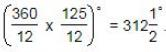Angle traced by minute hand in 25 min =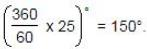∴ Reflex angle = 3600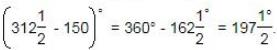QUESTION: 2

### Q. How many times in a day, the hands of a clock are straight?

Solution:

In 12 hours, the hands coincide or are in opposite direction 22 times.
∴ In 24 hours, the hands coincide or are in opposite direction 44 times a day.

QUESTION: 3

### A clock is started at noom. By 10 mimutes past 5, the hour hand has turned through:

Solution:

Angle traced by hour hand in 12 hrs = 360º.
Angle traced by hour hand in 5 hrs 10 min. i.e., 31/6 hrs
= (360/12*31/6)°
= 155º

QUESTION: 4

A watch which gains 5 seconds in 3 minutes was set right at 7 a.m. In the afternoon of the same day, when the watch indicated quarter past 4 o'clock, the true time is:

Solution:

Time from 7 a.m. to 4.15 p.m. = 9 hours 15 min =37/4 hours
3 min 5 sec of this clock = 3 min. of the correct clock.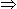37/720 hours of this clock =1/20 hours of the correct clock37hours of this clock =(1/20 x 720/37 x 37/4) hours of the correct clock.
= 9 hours of the correct clock.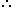The correct time is 9 hours after 7 a.m. i.e., 4 p.m

QUESTION: 5

At what time between 5.30 and 6 will the hands of a clock be at right angles?

Solution:

At 5 o'clock, the hands are 25 min. spaces apart.
To be at right angles and that too between 5.30 and 6, the minute hand has to gain (25 + 15) = 40 min. spaces.
55 min. spaces are gained in 60 min.
40 min. spaces are gained in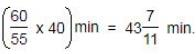∴ Required time =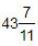min. past 5.

QUESTION: 6

At what time between 7 and 8 o'clock will the hands of a clock be in the same straight line but, not together?

Solution:

When the hands of the clock are in the same straight line but not together, they are 30 minute spaces apart.
At 7 o'clock, they are 25 min. spaces apart.Minute hand will have to gain only 5 min. spaces.
55 min. spaces are gained in 60 min.
5 min. spaces are gained in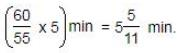∴ Required time =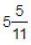min. past 7.

QUESTION: 7

A watch which gains uniformly is 2 minutes low at noon on Monday and is 4 min. 48 sec fast at 2 p.m. on the following Monday. When was it correct?

Solution:

Time from 12 p.m. on Monday to 2 p.m. on the following Monday = 7 days 2 hours = 170 hours.
∴ The watch gains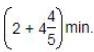in 170 hrs.
Now, 34/5 min. are gained in 170 hrs.
∴ 2 min. are gained in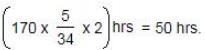Watch is correct 2 days 2 hrs. after 12 p.m. on Monday i.e., it will be correct at 2 p.m. on Wednesday.

QUESTION: 8

At what angle the hands of a clock are inclined at 15 minutes past 5?

Solution:

(11*15-60*5)/2=67.5

QUESTION: 9

The angle between the minute hand and the hour hand of a clock when the time is 4.20, is:

Solution:

The angle between hour and minute hand in 4:20 is 10 degrees.
For a minute, the hour hand rotates by 30/60 = 1/2 degrees.
hence, for 20 minutes it rotates by an angle of 20*1/2 = 10 degrees.

QUESTION: 10

A watch which gains uniformly is 2 minutes low at noon on Monday and is 4 min. 48 sec fast at 2 p.m. on the following Monday. When was it correct?

Solution:

Time from Monday noon (12 o' clock) to following Monday 2 pm = 7 day 2 hours
= 7×24+2
= 168+2
= 170 hours
Total time gained Monday noon (12 o' clock) to following Monday 2 pm = 2 min + 4 min 48 sec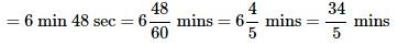The given watch gains 34/5 mins in 170 hours
Given that the said watch was 2 minutes low at Monday noon. Hence when it gained 2 minutes, the time was correct.
The given watch gains 34/5 mins in 170 hours
⇒ The given watch gains 2 mins in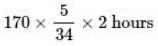= 5 * 5 * 2 hours = 50 hours
Hence the time was correct after 50 hours from Monday noon = after 2 days 2 hours from Monday noon = 2 pm on Wednesday